# AKU Previous Years Question Paper Solution

Q. State triangle law of forces and Lami’s theorem.

TRIANGLE LAW OF FORCE.

According to this law if there are three forces acting over a body which is in equilibrium. then, the two forces are represented as a two side of a triangle in same order with scaling their magnitude to a suitable scale then the third side or the closing side of the triangle would be the resultant in opposite order.Triangle law of forces

for example : let us take two forces of magnitude 50N and 100N acting on a same geometric plane at an angle of 30 ͦ and 60 ͦ respectively.

here, we can solve the following by triangle law of forces.first scale the forces into a suitable scale,then
step 1-from the point ‘o’ draw the first force(OA) value converted into scale at an angle of 30 ͦ made by the force with horizontal axis.
step 2- taking the first force (‘OA’) as the horizontal axis now draw the second force(‘AB’) at an angle of 60 ͦ made with the OA as axis.
step 3- now the point B is joined with the point O. The value or distance is calculated and then converted into Newton by multiplying the scale taken for drawing.

here the side of the triangle OB is the resultant of the two forces applied on a body without altering their effect on the body.

LAMI’S THEOREM.

This theorem was given by a Great Mathematician “Bernard Lamy”, and the name was coined Lami’s theorem.

According to this theorem, when three coplanar, concurrent and non-co-linear forces act on a body which is in equilibrium then the magnitude of each force is proportional to the sine of angle between other two forces.This theorem can be proved by the sine law.
( A/Sin α) = ( B/Sin β) = (C/ Sin γ)

also we can solve it by triangle law,proof of lami’s theorem

by the above empirical formulae we can calculate the magnitude of force on the body.

lami’s theorem?
Ans-when three coplanar, concurrent and non-co-linear forces act on a body which is in equilibrium then the magnitude of each force is proportional to the sine of angle between other two forces.

[AKU Paper Code 101101 Question No. 2 (A) 2019]

Q. If a vector of magnitude A is rotated through a certain degree, then what is the change in that vector? Explain.

A vector is represented by two quantities, magnitude and direction. Whatever be the angle of rotation, and whichever be the axis about which rotation is performed, the magnitude cannot change and remains invariant. It is the direction that changes. Since direction changes, the vector also gets changed.

[AKU Paper Code 101101 Question No. 2 (B) 2019]

Q. A projectile is launched from point with an initial speed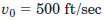directed as shown in the figure. Compute the x-, y-, and z-components of position, velocity, and acceleration 20 seconds after launch. Neglect aerodynamic drag.

V20= 500 sin6o = -433 ft/sec Vayo = 500 Costo = 250 ft/see V3 = 30-oft = 433-32.2420= -2 11 ft/sec Vay…

[AKU Paper Code 101101 Question No. 2 (C) 2019]

Q. Explain the conservative and non-conservative forces with examples.

##### Conservative Forces :
Conservative forces are those for which work done depends only on initial and final points.
For example, Gravitational Force, Electrostatic Force

##### Non-Conservative Forces :
Non-Conservative forces are those where the work done or the kinetic energy did depend on other factors such as the velocity or the particular path taken by the object.
For example, Frictional Force

[AKU Paper Code 101101 Question No. 3 (B) 2019]

Q. A flywheel of mass 8 tonnes starts from rest and gets up a speed of 180 r.p.m in 3 minutes. Find the average torque exerted on it, if the radius of gyration of the flywheel is 60cm.

[AKU Paper Code 101101 Question No. 4 (A) 2019]

Q. For the elliptical orbit of a spacecraft around the earth, determine the speed vA at point which results in a perigee altitude at of 200 km. What is the eccentricity of the orbit?

[AKU Paper Code 101101 Question No. 4 (B) 2019]

Q. How does Foucault pendulum. Explain Earth’s rotation?

[AKU Paper Code 101101 Question No. 5 (A) 2019]

Q. Describe the centripetal and Coriolis acceleration?

Centripetal acceleration, the acceleration of a body traversing a circular path. Because velocity is a vector quantity (that is, it has both a magnitude, the speed, and a direction), when a body travels on a circular path, its direction constantly changes and thus its velocity changes, producing an acceleration. The acceleration is directed radially toward the centre of the circle. The centripetal acceleration ac has a magnitude equal to the square of the body’s speed v along the curve divided by the distance r from the centre of the circle to the moving body; that is, ac = v2/r. Centripetal acceleration has units of metre per second squared. The force causing this acceleration is directed also toward the centre of the circle and is named centripetal force.

Coriolis force, also called Coriolis effect, in classical mechanics, an inertial force described by the 19th-century French engineer-mathematician Gustave-Gaspard Coriolis in 1835. Coriolis showed that, if the ordinary Newtonian laws of motion of bodies are to be used in a rotating frame of reference, an inertial force—acting to the right of the direction of body motion for counterclockwise rotation of the reference frame or to the left for clockwise rotation—must be included in the equations of motion.

[AKU Paper Code 101101 Question No. 5 (B) 2019]

Q. At the instant represented, the disk with the radial slot is rotating about O with a counterclockwise angular velocity if 4 rad/s, which is decreasing at a rate of 10 rad/s2. Motion of slider A in the slot is separately controlled, and at this instant, r = 150 mm, ṙ = 125 mm/s, r̈= 2025 mm/s2. Determine the absolute velocity and acceleration of A for this position.

[AKU Paper Code 101101 Question No. 5 (C) 2019]

Q. A body, moving with simple harmonic motion, has amplitude of 1 meter and the period of complete oscillation is 2 seconds. What will be the velocity and acceleration of the body after 0.4 seconds from the extreme position?

[AKU Paper Code 101101 Question No. 6 (A) 2019]

Q. Determine the amplitude of the steady-state motion of the 10-kg mass if (a)c=500 N.s/m and (b)c=0.

[AKU Paper Code 101101 Question No. 6 (B) 2019]

Q. Show that if the total linear momentum of a system of particles is zero, the angular momentum of the system is the same around all origins.

Given that the total linear momentum of the system of particles is zero,

i.e.,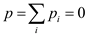We have to show that the angular momentum of the system is the same about all origins.

The total angular momentum is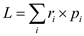, where,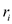is the vector from origin to point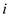.

The angular momentum generally depends on where the origin is.

We now want to find the angular momentum about a new origin, whose position vector is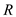, in the current coordinate system.

In the new system, the position vectorbecomes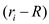.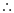The new value of angular momentum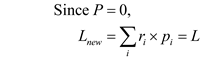Thus, if total linear momentum is zero, the angular momentum of the system is same about all origins.

[AKU Paper Code 101101 Question No. 7 (A) 2019]

Q. A particle with a mass of 4 kg has a position vector in meters given by r = 3t2i – 2tj -3tk, where is the time in seconds. For t = 3 s determine the magnitude of the angular momentum of the particle and the magnitude of the moment of all forces on the particle, both about the origin of coordinates.

[AKU Paper Code 101101 Question No. 7 (B) 2019]

Q. A mouse sits at the edge of a 40cm diameter record, rotating on a turntable at fifteen revolutions per minute. If the mouse walks straight inwards to a point 10cm from the center, what will be its new angular velocity? Assume the mass of the record is negligible.

[AKU Paper Code 101101 Question No. 8 (A) 2019]

Q. In the crank and connecting rod mechanism,the crank is 300mm long and the connecting rod is 1500mm long.If the crank rotates uniformly at 300 rpm. find the velocity of the cross head, when the crank is inclined at 30 degrees with the inner dead centre.

[AKU Paper Code 101101 Question No. 8 (B) 2019]

Q. The slender rod of mass and length rotates about the y-axis as the element of a right-circular cone. If the angular velocity about the y-axis is ω, determine the expression for the angular momentum of the rod with respect to the xyaxes for the particular position shown.

[AKU Paper Code 101101 Question No. 9 (A) 2019]

Q. A slender rod bent into the shape shown rotates about the fixed line CD at a constant angular rate ω. Determine the velocity and acceleration of point A.

[AKU Paper Code 101101 Question No. 9 (B) 2019]

we will update all solutions soon thanks for patience <3